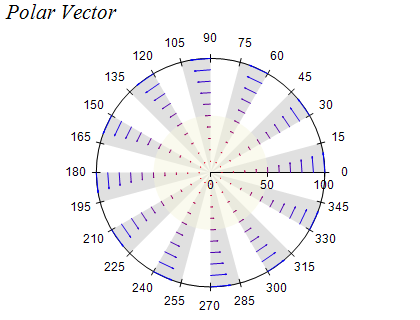﻿ Polar Vector Series
Nevron Open Vision Documentation
Chart / Charts / Types / Polar / Series / Polar Vector Series
In This Topic
Polar Vector Series
In This Topic

Polar Vector charts display vectors defined by two points (begin and end) which are represented as an arrow in polar coordinate space. An arrowhead is placed at the end point to indicate the vector direction. The following image shows a polar vector chart:Creating a Polar Vector Series

Polar Vector series are represented by the NPolarVectorSeries type. An instance of this type must be added to the series collection of a Polar chart:

C#
Copy Code

NPolarVectorSeries polarVector = new NPolarVectorSeries();

Passing Data

Polar Range series accept data points of type NPolarVectorDataPoint. The following code snippet shows how to add data to a polar vector series:

C#
Copy Code

NPolarVectorDataPoint polarVectorDataPoint = new NPolarVectorDataPoint();

polarVectorDataPoint.X = 0;

polarVectorDataPoint.Y = 0;

polarVectorDataPoint.X2 = 90;

polarVectorDataPoint.Y2 = 10;

The width and height of the vector arrowhead are relative to the vector length, so that shorter vectors have smaller arrowheads and longer vectors have larger arrowheads. The sizes are controlled through the ArrowheadSizeFactor properties. The MinArrowHeadSize and MaxArrowHeadSize properties can be used to set minimum and maximum sizes of the arrowhead.

Formatting Commands

The vector series supports the following formatting commands in addition to the standard (per data point) formatting commands:

<y1> - displays the vector y1 value. Has the same effect as the <value> command.
<x1> - displays the vector x1 values. Has the same effect as the <xvalue> command.

<x2> - displays the vector x2 value.
<y2> - displays the vector y2 value.